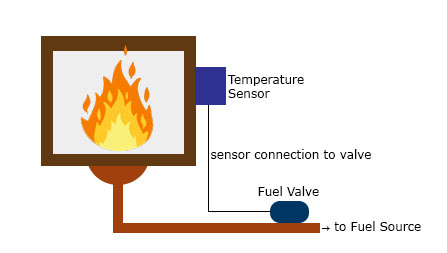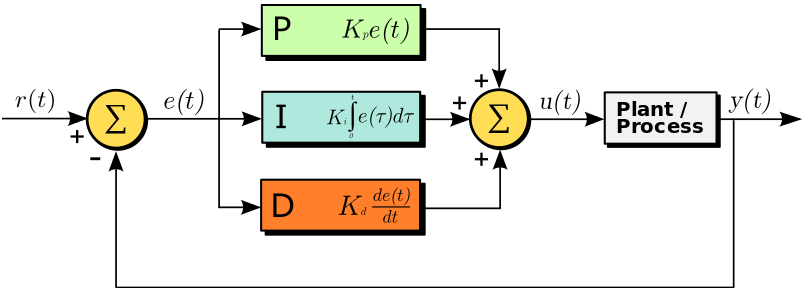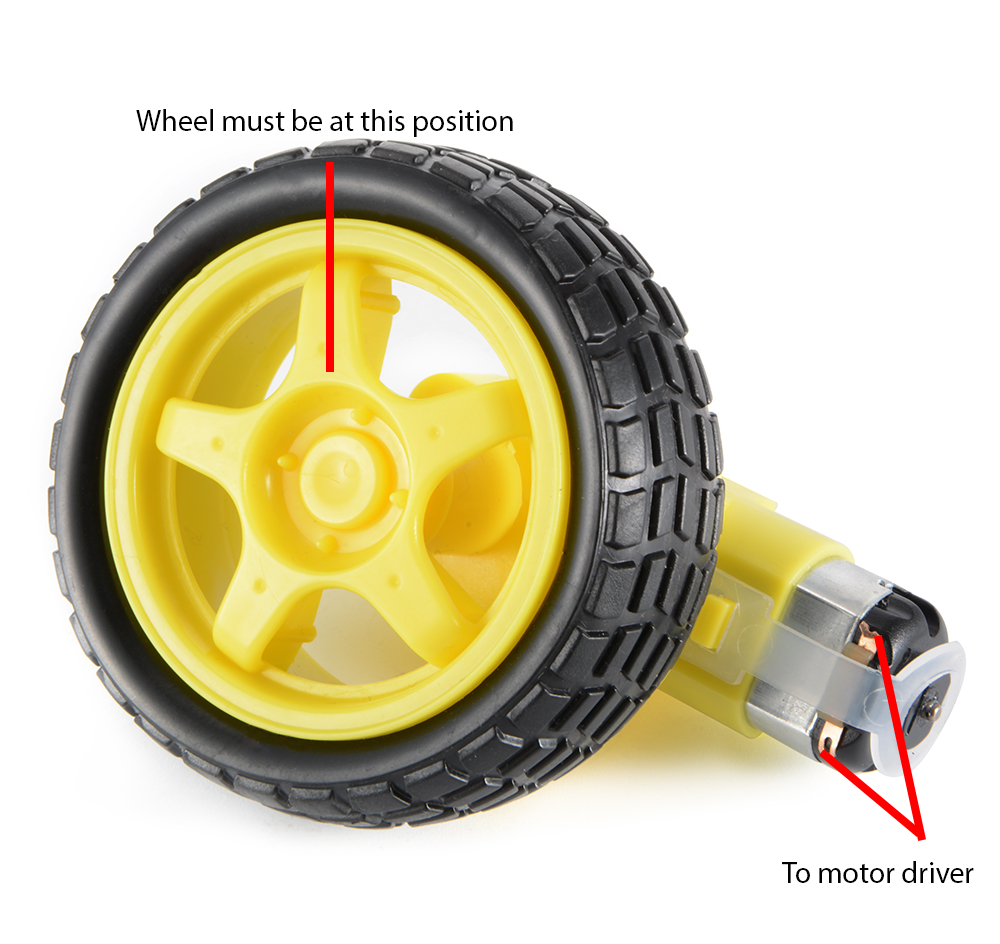Home / Tutorials / Arduino Tutorial / Arduino PID Control Tutorial# Arduino PID Control Tutorial

In control systems, a controller corrects the output of a particular system to a desired input in the presence of errors and disturbances. The most popular type of controller is PID which is an acronym for Proportional, Integral and Derivative. In this Arduino PID control tutorial, I will show you how you can employ such a controller in your project.

### What is PID?

As mentioned, PID is short for proportional, integral and derivative. The name comes from the methods on how such controller deals with disturbances in the system. However, such a controller is only in feedback systems. I suggest reading a material specifically written for such topic, but I’ll do my best to explain it here as simple as I can.

A feedback system is a system wherein part of the output is “fed back” to the input. For example, you could have a project that controls the fire in the furnace. Below is a simple illustration:You want to maintain the temperature in the furnace to a certain set point.  A sensor installed in the furnace determines the temperature at any time. This sensor, in this case, provides the feedback as a reference on the required temperature increase or decrease. The difference between the feedback sensor value and a temperature set point is the error.

#### Proportional Control

Proportional control refers to an adjustment that is proportional to how much the error is. Let’s say the controller in our example is an electronic valve for controlling the fuel to the furnace. If the error is small, the valve will release a small amount of fuel so that the set point and the feedback matches. If the error is large, the valve must release more fuel.

#### Integral Control

Proportional control produces offset in its correction due to disturbances. The Integral controller has the ability to remove this offset and bring back the error to zero. Such controller produces an adjustment that is based on the accumulated error over time. Without integral control, the system can’t deal with trends on errors.

Using our previous example, an offset may be present when the fuel valve didn’t return to its original position when it increased then decreased its fuel output. The integral controller will detect this, and will turn the fuel valve to its original position.

#### Derivative Control

Finally, Derivative control deals with the rate of change of the error. If integral control looks at the history of the error, derivative control predicts the error. Basically, the amount of correction will be based on how fast the error is changing. This type of controller works best with dynamic errors in which both proportional and integral controllers can’t deal with.

Let’s say the temperature in the furnace goes from 130 °C to 140 °C against a 120 °C set point in 2 seconds. The proportional and integral controllers will respond to the magnitude of the error, but it will have a hard time catching up to how fast the error occurred.. The derivative controller can deal with such because it has been looking at the rate of change of the error from the beginning.

A feedback system with a PID controller:Here the input variable or set point is r(t), output variable is y(t), controlled variable is u(t) and the error is e(t). Continuing with our furnace example, r(t) would be the desired temperature and y(t) is the actual temperature; e(t) is the difference between the desired temperature and actual temperature; u(t) is the sum of the corrections from the P, I and D controllers which is fed to the plant which is the fuel valve.

Note that a PID controller is not usable out of the box. Tuning must be done to ensure that the desired performance is achieved. This is done by carefully changing K constants as shown on the diagram above. These constants must be determined beforehand and changed according to the actual response of the system until the optimum values are achieved.

### Implementing PID in Code

To implement a PID controller in a code or an Arduino sketch, five parameters must be known: proportional, integral and derivative constants, input value and set point value.

PID computation must be inside a looping function. The first part of the function should be determining the time elapsed. In Arduino, the current time can be determined by millis() and the elapsed time is just:

```currentTime = millis();
elapsedTime = currentTime - previousTime;```

Next, the error must be determined:

`error = setPoint - input;`

Recall that the integral of the error is the cumulative error over time. To calculate an integral using Arduino, we simply do:

`cumError += error * elapsedTime;`

The derivative of the error is the rate of change of the error:

`rateError = (error - lastError)/elapsedTime;`

Finally, the computed output is:

`output = Kp * error + Ki * cumError + Kd * rateError;`

Here, the Kp, Ki and Kd are the predetermined constants.

Finally, the variables must be noted for the next iteration:

```lastError = error;
previousTime = currentTime;```

Let’s try a more concrete example. Imagine a wheel attached to a motor. We want the wheel to remain in the position shown:A rotary encoder on the wheel gives the angle in degrees of the current wheel position. At our desired wheel position, the angle is zero.

What we want is for the motor to turn whenever the wheel is out of position. Furthermore, the motor is controlled through pulse width modulation. The wider the pulse, the farther the motor rotates.

Next, let us implement this simple control system using an Arduino. Here is the sketch:

```//PID constants
double kp = 2
double ki = 5
double kd = 1

unsigned long currentTime, previousTime;
double elapsedTime;
double error;
double lastError;
double input, output, setPoint;
double cumError, rateError;

void setup(){
setPoint = 0;                          //set point at zero degrees
}

void loop(){
output = computePID(input);
delay(100);
analogWrite(3, output);                //control the motor based on PID value

}

double computePID(double inp){
currentTime = millis();                //get current time
elapsedTime = (double)(currentTime - previousTime);        //compute time elapsed from previous computation

error = Setpoint - inp;                                // determine error
cumError += error * elapsedTime;                // compute integral
rateError = (error - lastError)/elapsedTime;   // compute derivative

double out = kp*error + ki*cumError + kd*rateError;                //PID output

lastError = error;                                //remember current error
previousTime = currentTime;                        //remember current time

return out;                                        //have function return the PID output
}```

In the loop function, the rotary encoder determines the current position of the wheel and its output value becomes a parameter for the computePID() function. This function returns a value for controlling the motor using PWM.

### Arduino PID Library

We can further simplify the use of PID in Arduino projects with the help of Brett Beauregard’s PID library . The library only requires you to specify kd, ki, kp and setpoint values and you’re good to go!

Here is the PID_Basic.ino sketch that comes with the library. This sketch basically provides the same output as the sketch I provided above but better:

```#include <PID_v1.h>

#define PIN_INPUT 0
#define PIN_OUTPUT 3

//Define Variables we'll be connecting to
double Setpoint, Input, Output;

//Specify the links and initial tuning parameters
double Kp=2, Ki=5, Kd=1;
PID myPID(&Input, &Output, &Setpoint, Kp, Ki, Kd, DIRECT);

void setup()
{
//initialize the variables we're linked to
Setpoint = 100;

//turn the PID on
myPID.SetMode(AUTOMATIC);
}

void loop()
{
myPID.Compute();
analogWrite(PIN_OUTPUT, Output);
}```

Here, you can create a PID class and have the input, output, setpoint and k constants as parameters. To compute PID, simple call the Compute() function. It also contains a SetMode() function which turns on (AUTOMATIC) or turns off (MANUAL) the PID. The complete list of functions used by the library is found here.

I say that the sketch above is better than the one I provided is because it deals with PID limitations which is beyond the scope of this article.

### Closing

Hopefully, you learned about how to implement PID control in this article. I have used PID in a number of projects, including a self-balancing robot. If you have any questions about implementing Arduino PID, kindly drop a comment below!

## Arduino Pressure Sensor Tutorial | MPS20N0040D

This site has built up a number of tutorials on how to measure almost anything. …

1.for the code you provided and not the library , where do i write the value of my setpoint?

2.How to implement PID temperature control for MLX90614 IR sensor which gives reading I2C.

•Hi,

Get the temperature data and make it an input to the PID. The output of the PID is then used to control whatever that is generating heat on your project

•I WANT TO CONNECT A FAN IN CASE MY TEMP IS LESSER THAN THE SET POINT.WHAT CHANGING I SHOULD DO

3.Hi, I'm trying to balance a robot with one wheel using a stepper motor and accelerometer data that gives me current angle ... How can I implement the PID controller?

•Hello,

The basic idea would be that the input to your PID is the current angle of the robot while the output is the number of steps the stepper motor needs to move to counteract the offset of the robot. I’ve actually done a self balancing robot before but it was done using dc motors so I cant give anything more specific for your case.

•hello can i get the code for the self balancing robot? my robot would not give a feedback when it is turned on.

4.In your PID implementation (not the library) you use lastError before assignment, you should probably assign it a default value in setup just to be safe.

5.Hi,

My project is to control an actual which elongates by inflating or deflating air into. An amount of air is controlled by a velocity of air_pump. A distance is measured by a proximity sensor. So how can I use a PID algorithm to control the velocity of the air-pump, which is used to manipulate the actuator to a setpoint, based on a feedback value from the proximity sensor?

6.I have no idea about PID things. can you explain how could you get the value of KP, KI, and KD

7.Hi, nice explanation, thanks for that. I'm dealing with the following: in order to fire up (bake) ceramics, i need to gradually increase the temperature (let's say, going up to 650°C in about 6 hours). I like to use PID control to keep the temperature as close to the desired temperature as possible (on that point in time) but since i have to change (increase) the temperature gradually, the "set point" will keep changing over time. Do you think this would be possible? Raising the "set point" would result in an detected error thus "confusing" the derivative control, right? Or am i overthinking this a bit? 🙂 Thanks again. Cheers. p

•Hi,

That’s a good question. Actually, PID controller works with variable set points, as long as the variation is not random. Your example of gradually changing the set point temperature is a ramp type input, a common type of input in control systems. In your case, the error continuously changes because of the variation for both set point and actual temperature. The derivative term will deal with how fast that error is changing so there’s no problem there.

•8.Hi,

I am trying to build a differential drive robot(DDR) with DC motors with Arduino. The problem is that the built DDR does not move in a straight line because the two motors run at different speeds for the same input given by Pulse width Modulation.
Now, I had a plan to implement the PID controller in Arduino to control the DC motor. or to use a stepper motor or to use a Servo motor. Kindly give your opinion regarding this.

Thank you

9.How to determine the values of Kd, Kp, Ki??

10.Hello,

Thanks for the code. On this line:

analogWrite(3, output); //control the motor based on PID value

What is the 3 for?. Does it mean Pin 3?. Would it not have to be also defined as A3 or B1 or C0...etc??

On the other hand a ";" is missing after every PID constant at the beginning. "setpoint" is written with different capital letters. Just thought I would mention it for those who struggle because of those details detail.

•11.Hi,
To calculate the integral and derivative error, shouldn't we use the elapsed time in seconds instead of milliseconds?
I mean, dividing elapsedTime by 1000?

12.Is it possible to do multiple PID loop in single arduino code?

13.Very clear explanations.
Thanks a lot for the time you spend on this for us 😉

14.can you help me how to make coding to robot path tracer?i'm a newer for robotics project in my school

15.hi, assuming I have a 0 knowledge in PID, but were tasked to make a color sorter using PID in arduino, how do i code it and what are the tips or information i need to know? thankyou

16.hi, im trying to use PID library for a DC/DC boost converter but i kind confuse how to implement it.

17.when the dc motor reaches the specified set point the motor must stop. ie: the output must become zero, but if we use integral , when the error becomes zero, the cumulative error is still non zero thus making the output non zero hence the motor would still continue to run even after the set point is achieved. Correct me if I am wrong.

18.please how can implement PID controller with DHT sensor and dc fan motor

•Hi,

I assume that your input is temperature and output is the current to the fan. You need to establish a numerical relationship between the temperature and how much current is needed to drive the fan.

19.Nehemiah M.Kharpuri

Hi there, I have been trying to implement PID for regulating a PDLC film on the basis of temperature, I am using an ESP32 instead of an arduino. I am facing some problems with how to implement the same. Would be happy for your input

20.Hi,

I saw lot of videos about self balancing robots and I would like to build one. For this purpose I would use MPU-6050 and 2 DC motor with L298N motor driver and one Arduino Nano. I study vehicle engineering so I may say it is a little familier to me 😀 but also I could understand far better the concept if I could implement the PID algorithm. I know it would be easier to copy one of the solutions on the net but I want to do it for myself.
In previous comments I read You also bulit slef balancing robot. I would highly appreciate if You could help me and share some information and knowledge with me of your project. How you did it and what helped you to understand this?

Archi

21.Haha - bunch of folks who want you to write their code for them.
Thanks for the simple explanation.

22.Im trying to control an rlc circuit system with aurdino as an pid controller. I would like to know if I can give the variable set point to aurdino and how the output of aurdino be connected to be as in input to rlc circuit
Also can the aurdino be configured in discrete controller

23.Please send me the circuit diagram/schematic of temperature furnace.

24.the links have been Hacked and don't link to the expected material.

"list of function" and "limitations of PID"